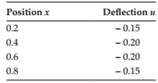Create an Account

Home / Questions / The deflection u of a beam along its longitudinal x axis has been recorded as shown in the...

The deflection u of a beam along its longitudinal x axis has been recorded as shown in the following table The bending moment at any point along this beam is modeled as

The deflection u of a beam along its longitudinal (x) axis has been recorded as shown in the following table. The bending moment at any point along this beam is modeled as M(x) = 1.05u″(x). All parameters are in consistent physical units. Find an estimate for the bending moment at x = 0.6 using

a. The three-point central difference formula

b. The three-point backward difference formulaJul 22 2020 View more View LessSubscribe To Get Solution